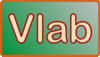Online V-notch Weir Calculators

131108

The programs online_vee_notch1 and online_vee_notch2 calculate the discharge through a V-notch weir (Fig. 1). Two types of V-notch weirs are considered: (1) a fully contracted weir, and (2) a partially contracted weir. A fully contracted weir is defined as that for which the ratio H/B ≤ 0.2. A partially contracted weir is that for which the ratio H/B ≤ 0.4.Fig. 1  V-notch weir schematic.

The program online_vee_notch1 is applicable to a fully contracted V-notch weir of angle θ in the range 25° ≤ θ ≤ 100°, while online_vee_notch2 is applicable to a partially contracted 90° V-notch weir. The computation procedures follow Section 7 of Chapter 7 of the USBR Water Measurement Manual.

Fully contracted vs partially contracted weir flow

Contraction refers to the size of the weir flow area as compared to the size of the approach channel flow area. Depending on size and design, a weir may be subject to both vertical and horizontal contraction. For a weir to be fully contracted, the ends of the weir should be sufficiently far from the sides and bottom of the approach channel. Full contraction increases the measurement accuracy by providing a more precise channel control (a unique discharge-stage relation) in the immediate vinicity of the weir.

For V-notch weirs, full contraction is produced when the distance b from each side of the weir notch to each side of the weir pool is greater than 2H. For a 90° V-notch weir, the flow width at head level is equal to 2H. Therefore, the weir may be considered to be fully contracted when the ratio B/H > 6, i.e., when H/B < 0.167. For a 60° notch weir, the requirement for a fully contracted weir is H/B < 0.194. In USBR practice, this translates into the practical criterion for a fully contracted V-notch weir: H/B ≤ 0.2.

A weir not satisfying the above criterion is partially contracted, i.e., the approach channel width B is too narrow relative to the head H. In USBR practice, the practical criterion for a partially contracted V-notch weir is: H/B ≤ 0.4.

Online V-notch calculator --- fully contracted

The fully contracted V-notch weir formula, due to Kindsvater and Shen (USBR Water Manual), is:

 Q = 4.28 Ce tan(θ/2) (H + k)5/2 

In Eq. 1, the discharge Q is a function of hydraulic head H and angle θ (radians). The discharge coefficient Ce is a function of θ (degrees). The head correction coefficient k is a funtion of θ (degrees). The width of the approach channel B is used to check to see if the weir is fully contracted:  H/B ≤ 0.2. Otherwise, the partially contracted weir calculator is recommended.

The formula (polinomial fit) for Ce, with θ in degrees, is:

Ce = 0.607165052 - 0.000874466963 θ + 0.0000061039334 θ2

The formula (polinomial fit) for k, with θ in degrees, is (LMNO Engineering):

k = 0.0144902648 - 0.00033955535 θ + 0.00000329819003 θ2 - 0.0000000106215442 θ3

The formula for the fully contracted V-notch weir is restricted to the following conditions:

1. Head H < 1.25 ft (38 cm).

2. Width B > 3 ft (91 cm).

3. Height P > 1.5 ft (46 cm).

4. Ratio b/H ≥ 2.0.

5. Head/width ratio H/B ≤ 0.2.

Online 90° V-notch calculator --- partially contracted

For the partially contracted 90° V-notch weir, the Kindsvater and Shen formula (Eq. 1) reduces to the following, in U.S. Customary units (Q in cfs, H in ft):

 Q = 4.28 Ce (H + 0.0029)5/2 

The discharge coefficient Ce is a function of the ratios H/P and P/B, as shown in Fig. 2.Source:  British Standard 3680, part 4A and ISO/TC 113/GT 2 (France-10) 1971.
Fig. 2  Variation of Ce as a function of H/P and P/B.

In online_vee_notch2, the discharge coefficient is obtained from Fig. 2 by digitization and discrete linear interpolation.

The formula for the partially contracted 90° V-notch weir is subject to the following restrictions:

1. Head H < 2 ft (61 cm).

2. Width B > 2 ft (61 cm).

3. Height P > 0.33 ft (10 cm).

4. Head/width ratio H/B ≤ 0.4.Fig. 1  V-notch weir schematic.

 131028